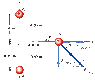# Find the magnitude and direction of force in a dipole

• kelkel

## Homework Statement

In Figure 21.12, two point charges q1=3uC and q2=-3uC interact with a third point charge Q=7uC. Find the magnitude and direction of the total (net) force on Q.

Edipole= kqs/r^3

## The Attempt at a Solution

I solved for the electric field with the above equation. I then multiplies that by the charge of Q to get the net force but it won't work! Help!

#### Attachments

•22-09alt.gif
5.3 KB · Views: 572